Successfully reported this slideshow.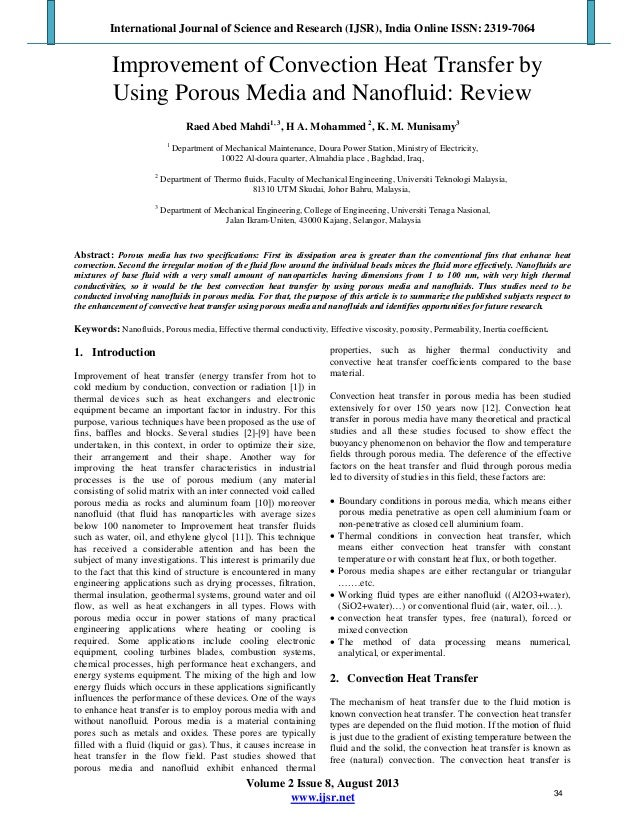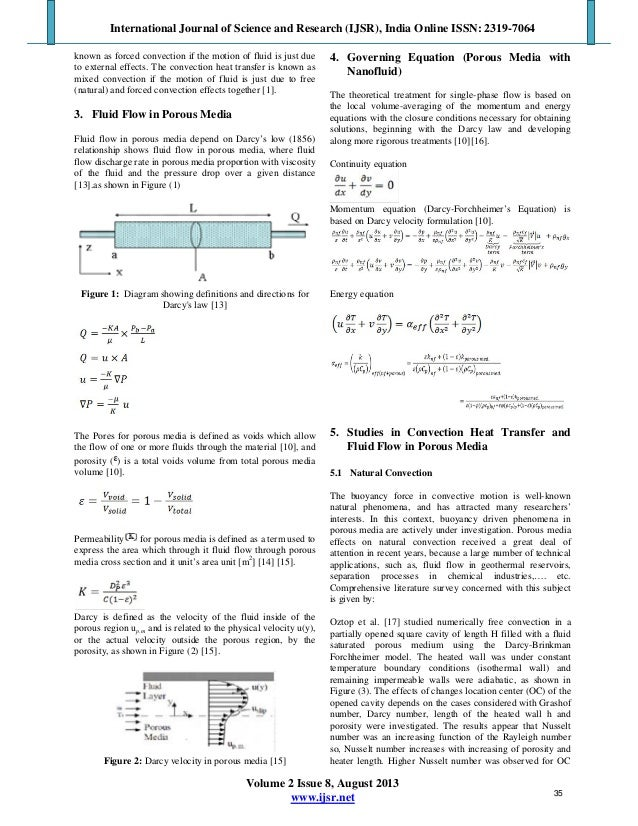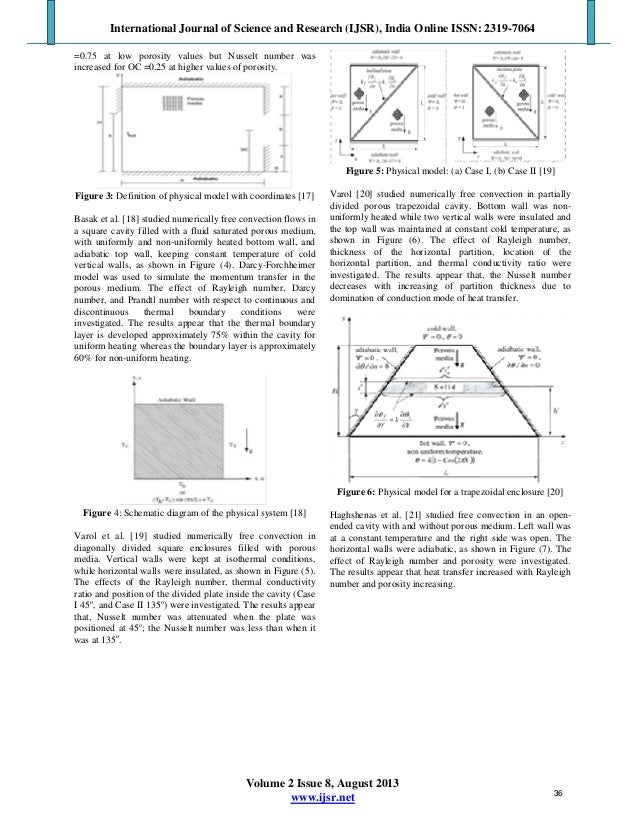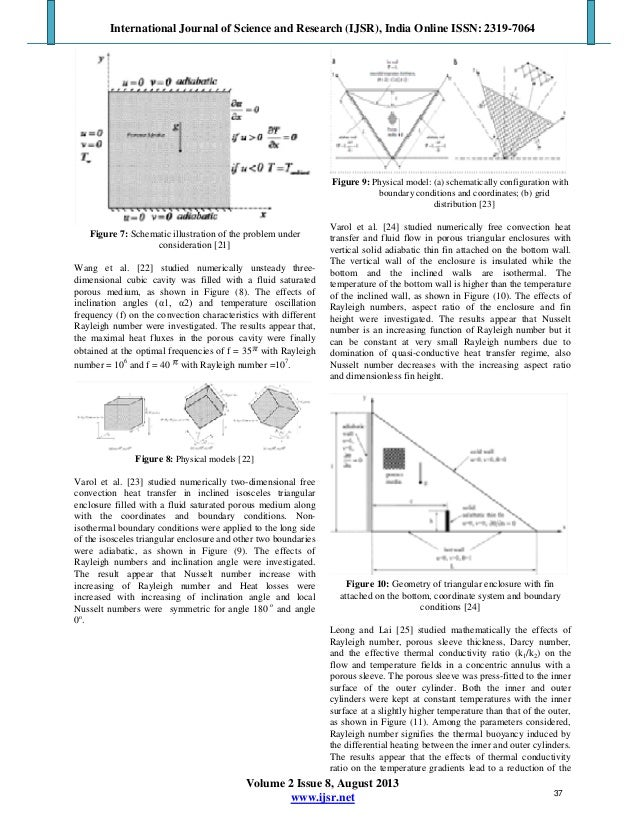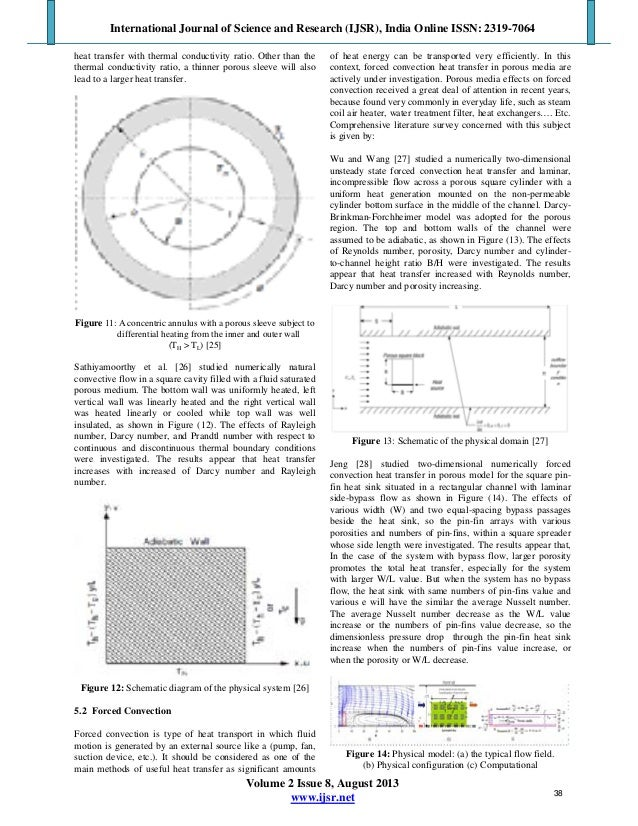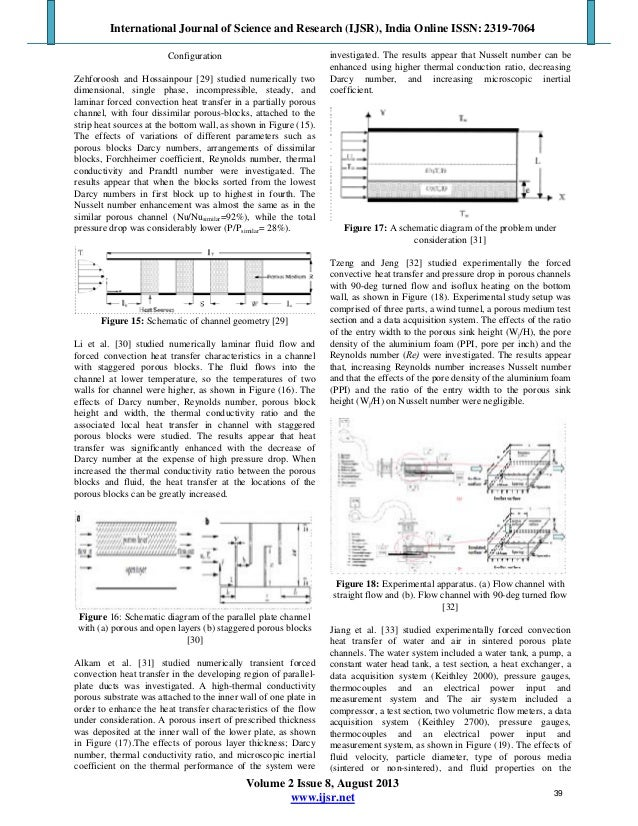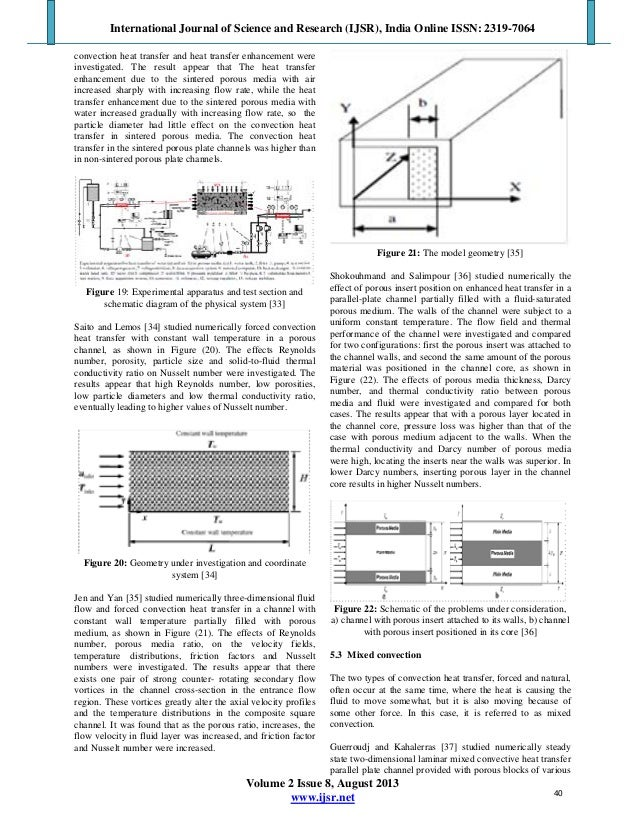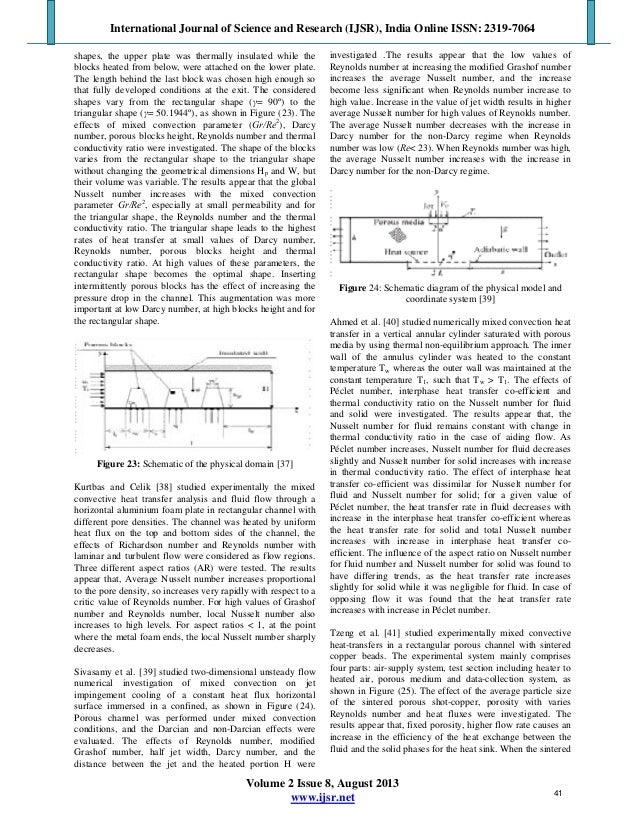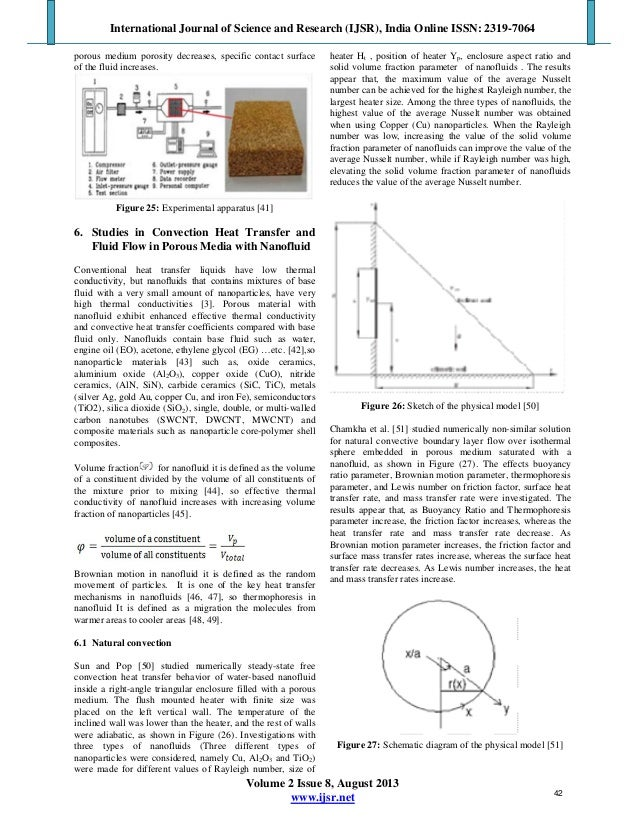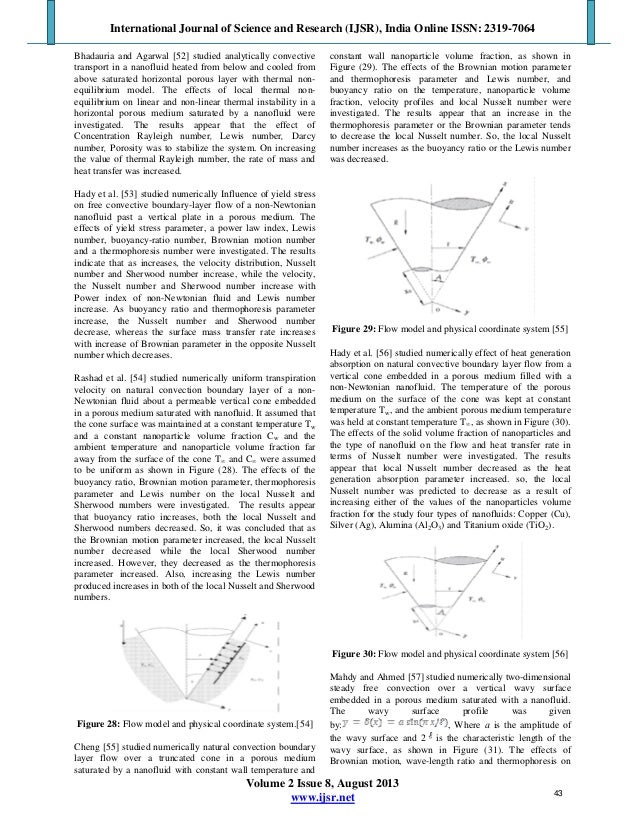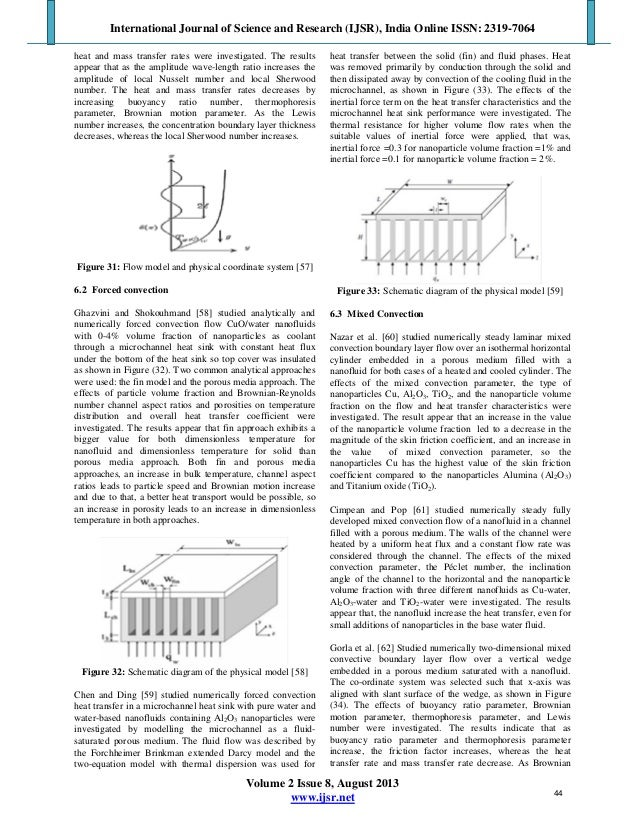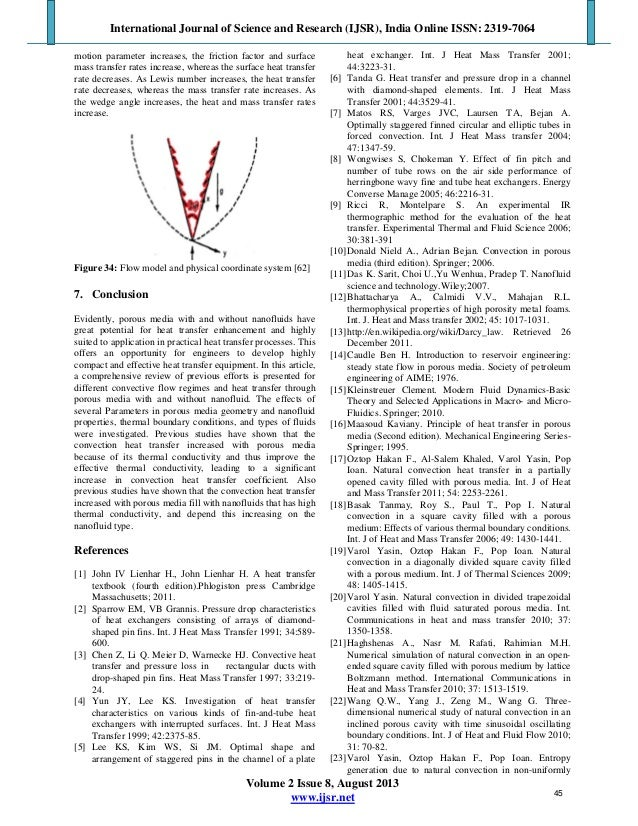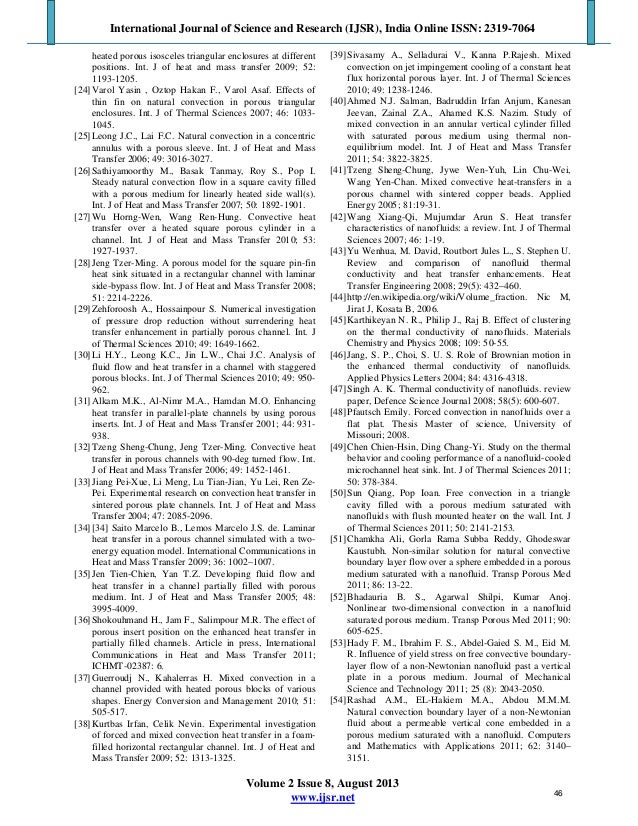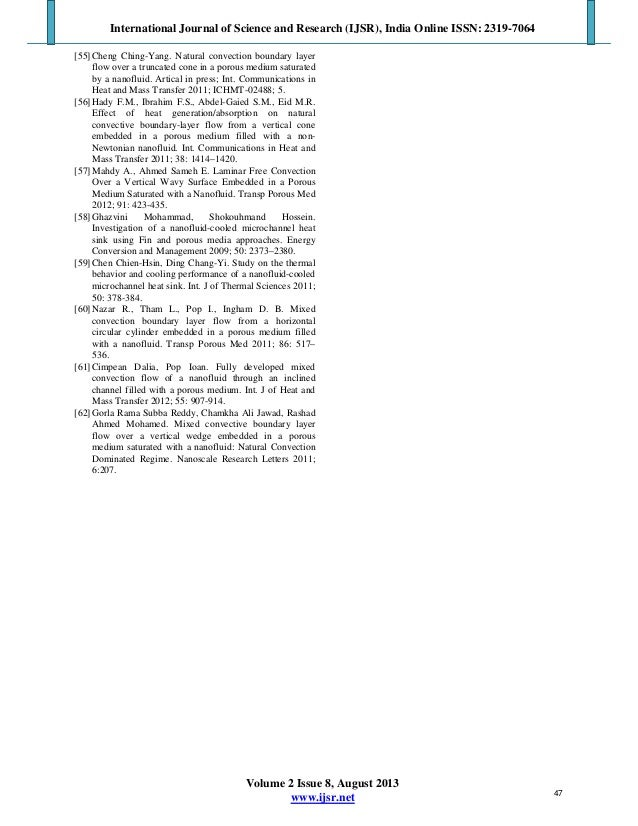Upcoming SlideShare
×

# Improvement of Convection Heat Transfer by Using Porous Media and Nanofluid: Review

Porous media has two specifications: First its dissipation area is greater than the conventional fins that enhance heat convection. Second the irregular motion of the fluid flow around the individual beads mixes the fluid more effectively. Nanofluids are mixtures of base fluid with a very small amount of nanoparticles having dimensions from 1 to 100 nm, with very high thermal conductivities, so it would be the best convection heat transfer by using porous media and nanofluids. Thus studies need to be conducted involving nanofluids in porous media. For that, the purpose of this article is to summarize the published subjects respect to the enhancement of convective heat transfer using porous media and nanofluids and identifies opportunities for future research.

• Full Name
Comment goes here.

Are you sure you want to Yes No• Be the first to comment

### Improvement of Convection Heat Transfer by Using Porous Media and Nanofluid: Review

1. 1. International Journal of Science and Research (IJSR), India Online ISSN: 2319-7064 Volume 2 Issue 8, August 2013 www.ijsr.net Improvement of Convection Heat Transfer by Using Porous Media and Nanofluid: Review Raed Abed Mahdi1, 3 , H A. Mohammed 2 , K. M. Munisamy3 1 Department of Mechanical Maintenance, Doura Power Station, Ministry of Electricity, 10022 Al-doura quarter, Almahdia place , Baghdad, Iraq, 2 Department of Thermo fluids, Faculty of Mechanical Engineering, Universiti Teknologi Malaysia, 81310 UTM Skudai, Johor Bahru, Malaysia, 3 Department of Mechanical Engineering, College of Engineering, Universiti Tenaga Nasional, Jalan Ikram-Uniten, 43000 Kajang, Selangor, Malaysia Abstract: Porous media has two specifications: First its dissipation area is greater than the conventional fins that enhance heat convection. Second the irregular motion of the fluid flow around the individual beads mixes the fluid more effectively. Nanofluids are mixtures of base fluid with a very small amount of nanoparticles having dimensions from 1 to 100 nm, with very high thermal conductivities, so it would be the best convection heat transfer by using porous media and nanofluids. Thus studies need to be conducted involving nanofluids in porous media. For that, the purpose of this article is to summarize the published subjects respect to the enhancement of convective heat transfer using porous media and nanofluids and identifies opportunities for future research. Keywords: Nanofluids, Porous media, Effective thermal conductivity, Effective viscosity, porosity, Permeability, Inertia coefficient. 1. Introduction Improvement of heat transfer (energy transfer from hot to cold medium by conduction, convection or radiation ) in thermal devices such as heat exchangers and electronic equipment became an important factor in industry. For this purpose, various techniques have been proposed as the use of fins, baffles and blocks. Several studies - have been undertaken, in this context, in order to optimize their size, their arrangement and their shape. Another way for improving the heat transfer characteristics in industrial processes is the use of porous medium (any material consisting of solid matrix with an inter connected void called porous media as rocks and aluminum foam ) moreover nanofluid (that fluid has nanoparticles with average sizes below 100 nanometer to Improvement heat transfer fluids such as water, oil, and ethylene glycol ). This technique has received a considerable attention and has been the subject of many investigations. This interest is primarily due to the fact that this kind of structure is encountered in many engineering applications such as drying processes, filtration, thermal insulation, geothermal systems, ground water and oil flow, as well as heat exchangers in all types. Flows with porous media occur in power stations of many practical engineering applications where heating or cooling is required. Some applications include cooling electronic equipment, cooling turbines blades, combustion systems, chemical processes, high performance heat exchangers, and energy systems equipment. The mixing of the high and low energy fluids which occurs in these applications significantly influences the performance of these devices. One of the ways to enhance heat transfer is to employ porous media with and without nanofluid. Porous media is a material containing pores such as metals and oxides. These pores are typically filled with a fluid (liquid or gas). Thus, it causes increase in heat transfer in the flow field. Past studies showed that porous media and nanofluid exhibit enhanced thermal properties, such as higher thermal conductivity and convective heat transfer coefficients compared to the base material. Convection heat transfer in porous media has been studied extensively for over 150 years now . Convection heat transfer in porous media have many theoretical and practical studies and all these studies focused to show effect the buoyancy phenomenon on behavior the flow and temperature fields through porous media. The deference of the effective factors on the heat transfer and fluid through porous media led to diversity of studies in this field, these factors are: • Boundary conditions in porous media, which means either porous media penetrative as open cell aluminium foam or non-penetrative as closed cell aluminium foam. • Thermal conditions in convection heat transfer, which means either convection heat transfer with constant temperature or with constant heat flux, or both together. • Porous media shapes are either rectangular or triangular ….…etc. • Working fluid types are either nanofluid ((Al2O3+water), (SiO2+water)…) or conventional fluid (air, water, oil…). • convection heat transfer types, free (natural), forced or mixed convection • The method of data processing means numerical, analytical, or experimental. 2. Convection Heat Transfer The mechanism of heat transfer due to the fluid motion is known convection heat transfer. The convection heat transfer types are depended on the fluid motion. If the motion of fluid is just due to the gradient of existing temperature between the fluid and the solid, the convection heat transfer is known as free (natural) convection. The convection heat transfer is 34
2. 2. International Journal of Science and Research (IJSR), India Online ISSN: 2319-7064 Volume 2 Issue 8, August 2013 www.ijsr.net known as forced convection if the motion of fluid is just due to external effects. The convection heat transfer is known as mixed convection if the motion of fluid is just due to free (natural) and forced convection effects together . 3. Fluid Flow in Porous Media Fluid flow in porous media depend on Darcy’s low (1856) relationship shows fluid flow in porous media, where fluid flow discharge rate in porous media proportion with viscosity of the fluid and the pressure drop over a given distance .as shown in Figure (1) Figure 1: Diagram showing definitions and directions for Darcy's law  The Pores for porous media is defined as voids which allow the flow of one or more fluids through the material , and porosity ( ) is a total voids volume from total porous media volume . Permeability for porous media is defined as a term used to express the area which through it fluid flow through porous media cross section and it unit’s area unit [m2 ]  . Darcy is defined as the velocity of the fluid inside of the porous region up.m and is related to the physical velocity u(y), or the actual velocity outside the porous region, by the porosity, as shown in Figure (2) . Figure 2: Darcy velocity in porous media  4. Governing Equation (Porous Media with Nanofluid) The theoretical treatment for single-phase flow is based on the local volume-averaging of the momentum and energy equations with the closure conditions necessary for obtaining solutions, beginning with the Darcy law and developing along more rigorous treatments . Continuity equation Momentum equation (Darcy-Forchheimer’s Equation) is based on Darcy velocity formulation . Energy equation 5. Studies in Convection Heat Transfer and Fluid Flow in Porous Media 5.1 Natural Convection The buoyancy force in convective motion is well-known natural phenomena, and has attracted many researchers’ interests. In this context, buoyancy driven phenomena in porous media are actively under investigation. Porous media effects on natural convection received a great deal of attention in recent years, because a large number of technical applications, such as, fluid flow in geothermal reservoirs, separation processes in chemical industries,…. etc. Comprehensive literature survey concerned with this subject is given by: Oztop et al.  studied numerically free convection in a partially opened square cavity of length H filled with a fluid saturated porous medium using the Darcy-Brinkman Forchheimer model. The heated wall was under constant temperature boundary conditions (isothermal wall) and remaining impermeable walls were adiabatic, as shown in Figure (3). The effects of changes location center (OC) of the opened cavity depends on the cases considered with Grashof number, Darcy number, length of the heated wall h and porosity were investigated. The results appear that Nusselt number was an increasing function of the Rayleigh number so, Nusselt number increases with increasing of porosity and heater length. Higher Nusselt number was observed for OC 35
3. 3. International Journal of Science and Research (IJSR), India Online ISSN: 2319-7064 Volume 2 Issue 8, August 2013 www.ijsr.net =0.75 at low porosity values but Nusselt number was increased for OC =0.25 at higher values of porosity. Figure 3: Definition of physical model with coordinates  Basak et al.  studied numerically free convection flows in a square cavity filled with a fluid saturated porous medium, with uniformly and non-uniformly heated bottom wall, and adiabatic top wall, keeping constant temperature of cold vertical walls, as shown in Figure (4). Darcy-Forchheimer model was used to simulate the momentum transfer in the porous medium. The effect of Rayleigh number, Darcy number, and Prandtl number with respect to continuous and discontinuous thermal boundary conditions were investigated. The results appear that the thermal boundary layer is developed approximately 75% within the cavity for uniform heating whereas the boundary layer is approximately 60% for non-uniform heating. Figure 4: Schematic diagram of the physical system  Varol et al.  studied numerically free convection in diagonally divided square enclosures filled with porous media. Vertical walls were kept at isothermal conditions, while horizontal walls were insulated, as shown in Figure (5). The effects of the Rayleigh number, thermal conductivity ratio and position of the divided plate inside the cavity (Case I 45ο , and Case II 135ο ) were investigated. The results appear that, Nusselt number was attenuated when the plate was positioned at 45ο ; the Nusselt number was less than when it was at 135ο . Figure 5: Physical model: (a) Case I, (b) Case II  Varol  studied numerically free convection in partially divided porous trapezoidal cavity. Bottom wall was non- uniformly heated while two vertical walls were insulated and the top wall was maintained at constant cold temperature, as shown in Figure (6). The effect of Rayleigh number, thickness of the horizontal partition, location of the horizontal partition, and thermal conductivity ratio were investigated. The results appear that, the Nusselt number decreases with increasing of partition thickness due to domination of conduction mode of heat transfer. Figure 6: Physical model for a trapezoidal enclosure  Haghshenas et al.  studied free convection in an open- ended cavity with and without porous medium. Left wall was at a constant temperature and the right side was open. The horizontal walls were adiabatic, as shown in Figure (7). The effect of Rayleigh number and porosity were investigated. The results appear that heat transfer increased with Rayleigh number and porosity increasing. 36
4. 4. International Journal of Science and Research (IJSR), India Online ISSN: 2319-7064 Volume 2 Issue 8, August 2013 www.ijsr.net Figure 7: Schematic illustration of the problem under consideration  Wang et al.  studied numerically unsteady three- dimensional cubic cavity was filled with a fluid saturated porous medium, as shown in Figure (8). The effects of inclination angles (α1, α2) and temperature oscillation frequency (f) on the convection characteristics with different Rayleigh number were investigated. The results appear that, the maximal heat fluxes in the porous cavity were finally obtained at the optimal frequencies of f = 35 with Rayleigh number = 106 and f = 40 with Rayleigh number =107 . Figure 8: Physical models  Varol et al.  studied numerically two-dimensional free convection heat transfer in inclined isosceles triangular enclosure filled with a fluid saturated porous medium along with the coordinates and boundary conditions. Non- isothermal boundary conditions were applied to the long side of the isosceles triangular enclosure and other two boundaries were adiabatic, as shown in Figure (9). The effects of Rayleigh numbers and inclination angle were investigated. The result appear that Nusselt number increase with increasing of Rayleigh number and Heat losses were increased with increasing of inclination angle and local Nusselt numbers were symmetric for angle 180 ο and angle 0ο . Figure 9: Physical model: (a) schematically configuration with boundary conditions and coordinates; (b) grid distribution  Varol et al.  studied numerically free convection heat transfer and fluid flow in porous triangular enclosures with vertical solid adiabatic thin fin attached on the bottom wall. The vertical wall of the enclosure is insulated while the bottom and the inclined walls are isothermal. The temperature of the bottom wall is higher than the temperature of the inclined wall, as shown in Figure (10). The effects of Rayleigh numbers, aspect ratio of the enclosure and fin height were investigated. The results appear that Nusselt number is an increasing function of Rayleigh number but it can be constant at very small Rayleigh numbers due to domination of quasi-conductive heat transfer regime, also Nusselt number decreases with the increasing aspect ratio and dimensionless fin height. Figure 10: Geometry of triangular enclosure with fin attached on the bottom, coordinate system and boundary conditions  Leong and Lai  studied mathematically the effects of Rayleigh number, porous sleeve thickness, Darcy number, and the effective thermal conductivity ratio (k1/k2) on the flow and temperature fields in a concentric annulus with a porous sleeve. The porous sleeve was press-fitted to the inner surface of the outer cylinder. Both the inner and outer cylinders were kept at constant temperatures with the inner surface at a slightly higher temperature than that of the outer, as shown in Figure (11). Among the parameters considered, Rayleigh number signifies the thermal buoyancy induced by the differential heating between the inner and outer cylinders. The results appear that the effects of thermal conductivity ratio on the temperature gradients lead to a reduction of the 37
5. 5. International Journal of Science and Research (IJSR), India Online ISSN: 2319-7064 Volume 2 Issue 8, August 2013 www.ijsr.net heat transfer with thermal conductivity ratio. Other than the thermal conductivity ratio, a thinner porous sleeve will also lead to a larger heat transfer. Figure 11: A concentric annulus with a porous sleeve subject to differential heating from the inner and outer wall (TH > TL)  Sathiyamoorthy et al.  studied numerically natural convective flow in a square cavity filled with a fluid saturated porous medium. The bottom wall was uniformly heated, left vertical wall was linearly heated and the right vertical wall was heated linearly or cooled while top wall was well insulated, as shown in Figure (12). The effects of Rayleigh number, Darcy number, and Prandtl number with respect to continuous and discontinuous thermal boundary conditions were investigated. The results appear that heat transfer increases with increased of Darcy number and Rayleigh number. Figure 12: Schematic diagram of the physical system  5.2 Forced Convection Forced convection is type of heat transport in which fluid motion is generated by an external source like a (pump, fan, suction device, etc.). It should be considered as one of the main methods of useful heat transfer as significant amounts of heat energy can be transported very efficiently. In this context, forced convection heat transfer in porous media are actively under investigation. Porous media effects on forced convection received a great deal of attention in recent years, because found very commonly in everyday life, such as steam coil air heater, water treatment filter, heat exchangers…. Etc. Comprehensive literature survey concerned with this subject is given by: Wu and Wang  studied a numerically two-dimensional unsteady state forced convection heat transfer and laminar, incompressible flow across a porous square cylinder with a uniform heat generation mounted on the non-permeable cylinder bottom surface in the middle of the channel. Darcy- Brinkman-Forchheimer model was adopted for the porous region. The top and bottom walls of the channel were assumed to be adiabatic, as shown in Figure (13). The effects of Reynolds number, porosity, Darcy number and cylinder- to-channel height ratio B/H were investigated. The results appear that heat transfer increased with Reynolds number, Darcy number and porosity increasing. Figure 13: Schematic of the physical domain  Jeng  studied two-dimensional numerically forced convection heat transfer in porous model for the square pin- fin heat sink situated in a rectangular channel with laminar side-bypass flow as shown in Figure (14). The effects of various width (W) and two equal-spacing bypass passages beside the heat sink, so the pin-fin arrays with various porosities and numbers of pin-fins, within a square spreader whose side length were investigated. The results appear that, In the case of the system with bypass flow, larger porosity promotes the total heat transfer, especially for the system with larger W/L value. But when the system has no bypass flow, the heat sink with same numbers of pin-fins value and various e will have the similar the average Nusselt number. The average Nusselt number decrease as the W/L value increase or the numbers of pin-fins value decrease, so the dimensionless pressure drop through the pin-fin heat sink increase when the numbers of pin-fins value increase, or when the porosity or W/L decrease. Figure 14: Physical model: (a) the typical flow field. (b) Physical configuration (c) Computational 38
6. 6. International Journal of Science and Research (IJSR), India Online ISSN: 2319-7064 Volume 2 Issue 8, August 2013 www.ijsr.net Configuration Zehforoosh and Hossainpour  studied numerically two dimensional, single phase, incompressible, steady, and laminar forced convection heat transfer in a partially porous channel, with four dissimilar porous-blocks, attached to the strip heat sources at the bottom wall, as shown in Figure (15). The effects of variations of different parameters such as porous blocks Darcy numbers, arrangements of dissimilar blocks, Forchheimer coefficient, Reynolds number, thermal conductivity and Prandtl number were investigated. The results appear that when the blocks sorted from the lowest Darcy numbers in first block up to highest in fourth. The Nusselt number enhancement was almost the same as in the similar porous channel (Nu/Nusimilar=92%), while the total pressure drop was considerably lower (P/Psimilar= 28%). Figure 15: Schematic of channel geometry  Li et al.  studied numerically laminar fluid flow and forced convection heat transfer characteristics in a channel with staggered porous blocks. The fluid flows into the channel at lower temperature, so the temperatures of two walls for channel were higher, as shown in Figure (16). The effects of Darcy number, Reynolds number, porous block height and width, the thermal conductivity ratio and the associated local heat transfer in channel with staggered porous blocks were studied. The results appear that heat transfer was significantly enhanced with the decrease of Darcy number at the expense of high pressure drop. When increased the thermal conductivity ratio between the porous blocks and fluid, the heat transfer at the locations of the porous blocks can be greatly increased. Figure 16: Schematic diagram of the parallel plate channel with (a) porous and open layers (b) staggered porous blocks  Alkam et al.  studied numerically transient forced convection heat transfer in the developing region of parallel- plate ducts was investigated. A high-thermal conductivity porous substrate was attached to the inner wall of one plate in order to enhance the heat transfer characteristics of the flow under consideration. A porous insert of prescribed thickness was deposited at the inner wall of the lower plate, as shown in Figure (17).The effects of porous layer thickness; Darcy number, thermal conductivity ratio, and microscopic inertial coefficient on the thermal performance of the system were investigated. The results appear that Nusselt number can be enhanced using higher thermal conduction ratio, decreasing Darcy number, and increasing microscopic inertial coefficient. Figure 17: A schematic diagram of the problem under consideration  Tzeng and Jeng  studied experimentally the forced convective heat transfer and pressure drop in porous channels with 90-deg turned flow and isoflux heating on the bottom wall, as shown in Figure (18). Experimental study setup was comprised of three parts, a wind tunnel, a porous medium test section and a data acquisition system. The effects of the ratio of the entry width to the porous sink height (Wj/H), the pore density of the aluminium foam (PPI, pore per inch) and the Reynolds number (Re) were investigated. The results appear that, increasing Reynolds number increases Nusselt number and that the effects of the pore density of the aluminium foam (PPI) and the ratio of the entry width to the porous sink height (Wj/H) on Nusselt number were negligible. Figure 18: Experimental apparatus. (a) Flow channel with straight flow and (b). Flow channel with 90-deg turned flow  Jiang et al.  studied experimentally forced convection heat transfer of water and air in sintered porous plate channels. The water system included a water tank, a pump, a constant water head tank, a test section, a heat exchanger, a data acquisition system (Keithley 2000), pressure gauges, thermocouples and an electrical power input and measurement system and The air system included a compressor, a test section, two volumetric flow meters, a data acquisition system (Keithley 2700), pressure gauges, thermocouples and an electrical power input and measurement system, as shown in Figure (19). The effects of fluid velocity, particle diameter, type of porous media (sintered or non-sintered), and fluid properties on the 39
7. 7. International Journal of Science and Research (IJSR), India Online ISSN: 2319-7064 Volume 2 Issue 8, August 2013 www.ijsr.net convection heat transfer and heat transfer enhancement were investigated. The result appear that The heat transfer enhancement due to the sintered porous media with air increased sharply with increasing flow rate, while the heat transfer enhancement due to the sintered porous media with water increased gradually with increasing flow rate, so the particle diameter had little effect on the convection heat transfer in sintered porous media. The convection heat transfer in the sintered porous plate channels was higher than in non-sintered porous plate channels. Figure 19: Experimental apparatus and test section and schematic diagram of the physical system  Saito and Lemos  studied numerically forced convection heat transfer with constant wall temperature in a porous channel, as shown in Figure (20). The effects Reynolds number, porosity, particle size and solid-to-fluid thermal conductivity ratio on Nusselt number were investigated. The results appear that high Reynolds number, low porosities, low particle diameters and low thermal conductivity ratio, eventually leading to higher values of Nusselt number. Figure 20: Geometry under investigation and coordinate system  Jen and Yan  studied numerically three-dimensional fluid flow and forced convection heat transfer in a channel with constant wall temperature partially filled with porous medium, as shown in Figure (21). The effects of Reynolds number, porous media ratio, on the velocity fields, temperature distributions, friction factors and Nusselt numbers were investigated. The results appear that there exists one pair of strong counter- rotating secondary flow vortices in the channel cross-section in the entrance flow region. These vortices greatly alter the axial velocity profiles and the temperature distributions in the composite square channel. It was found that as the porous ratio, increases, the flow velocity in fluid layer was increased, and friction factor and Nusselt number were increased. Figure 21: The model geometry  Shokouhmand and Salimpour  studied numerically the effect of porous insert position on enhanced heat transfer in a parallel-plate channel partially filled with a fluid-saturated porous medium. The walls of the channel were subject to a uniform constant temperature. The flow field and thermal performance of the channel were investigated and compared for two configurations: first the porous insert was attached to the channel walls, and second the same amount of the porous material was positioned in the channel core, as shown in Figure (22). The effects of porous media thickness, Darcy number, and thermal conductivity ratio between porous media and fluid were investigated and compared for both cases. The results appear that with a porous layer located in the channel core, pressure loss was higher than that of the case with porous medium adjacent to the walls. When the thermal conductivity and Darcy number of porous media were high, locating the inserts near the walls was superior. In lower Darcy numbers, inserting porous layer in the channel core results in higher Nusselt numbers. Figure 22: Schematic of the problems under consideration, a) channel with porous insert attached to its walls, b) channel with porous insert positioned in its core  5.3 Mixed convection The two types of convection heat transfer, forced and natural, often occur at the same time, where the heat is causing the fluid to move somewhat, but it is also moving because of some other force. In this case, it is referred to as mixed convection. Guerroudj and Kahalerras  studied numerically steady state two-dimensional laminar mixed convective heat transfer parallel plate channel provided with porous blocks of various 40
8. 8. International Journal of Science and Research (IJSR), India Online ISSN: 2319-7064 Volume 2 Issue 8, August 2013 www.ijsr.net shapes, the upper plate was thermally insulated while the blocks heated from below, were attached on the lower plate. The length behind the last block was chosen high enough so that fully developed conditions at the exit. The considered shapes vary from the rectangular shape (γ= 90º) to the triangular shape (γ= 50.1944º), as shown in Figure (23). The effects of mixed convection parameter (Gr/Re2 ), Darcy number, porous blocks height, Reynolds number and thermal conductivity ratio were investigated. The shape of the blocks varies from the rectangular shape to the triangular shape without changing the geometrical dimensions Hp and W, but their volume was variable. The results appear that the global Nusselt number increases with the mixed convection parameter Gr/Re2 , especially at small permeability and for the triangular shape, the Reynolds number and the thermal conductivity ratio. The triangular shape leads to the highest rates of heat transfer at small values of Darcy number, Reynolds number, porous blocks height and thermal conductivity ratio. At high values of these parameters, the rectangular shape becomes the optimal shape. Inserting intermittently porous blocks has the effect of increasing the pressure drop in the channel. This augmentation was more important at low Darcy number, at high blocks height and for the rectangular shape. Figure 23: Schematic of the physical domain  Kurtbas and Celik  studied experimentally the mixed convective heat transfer analysis and fluid flow through a horizontal aluminium foam plate in rectangular channel with different pore densities. The channel was heated by uniform heat flux on the top and bottom sides of the channel, the effects of Richardson number and Reynolds number with laminar and turbulent flow were considered as flow regions. Three different aspect ratios (AR) were tested. The results appear that, Average Nusselt number increases proportional to the pore density, so increases very rapidly with respect to a critic value of Reynolds number. For high values of Grashof number and Reynolds number, local Nusselt number also increases to high levels. For aspect ratios < 1, at the point where the metal foam ends, the local Nusselt number sharply decreases. Sivasamy et al.  studied two-dimensional unsteady flow numerical investigation of mixed convection on jet impingement cooling of a constant heat flux horizontal surface immersed in a confined, as shown in Figure (24). Porous channel was performed under mixed convection conditions, and the Darcian and non-Darcian effects were evaluated. The effects of Reynolds number, modified Grashof number, half jet width, Darcy number, and the distance between the jet and the heated portion H were investigated .The results appear that the low values of Reynolds number at increasing the modified Grashof number increases the average Nusselt number, and the increase become less significant when Reynolds number increase to high value. Increase in the value of jet width results in higher average Nusselt number for high values of Reynolds number. The average Nusselt number decreases with the increase in Darcy number for the non-Darcy regime when Reynolds number was low (Re< 23). When Reynolds number was high, the average Nusselt number increases with the increase in Darcy number for the non-Darcy regime. Figure 24: Schematic diagram of the physical model and coordinate system  Ahmed et al.  studied numerically mixed convection heat transfer in a vertical annular cylinder saturated with porous media by using thermal non-equilibrium approach. The inner wall of the annulus cylinder was heated to the constant temperature Tw whereas the outer wall was maintained at the constant temperature T1, such that Tw > T1. The effects of Péclet number, interphase heat transfer co-efficient and thermal conductivity ratio on the Nusselt number for fluid and solid were investigated. The results appear that, the Nusselt number for fluid remains constant with change in thermal conductivity ratio in the case of aiding flow. As Péclet number increases, Nusselt number for fluid decreases slightly and Nusselt number for solid increases with increase in thermal conductivity ratio. The effect of interphase heat transfer co-efficient was dissimilar for Nusselt number for fluid and Nusselt number for solid; for a given value of Péclet number, the heat transfer rate in fluid decreases with increase in the interphase heat transfer co-efficient whereas the heat transfer rate for solid and total Nusselt number increases with increase in interphase heat transfer co- efficient. The influence of the aspect ratio on Nusselt number for fluid number and Nusselt number for solid was found to have differing trends, as the heat transfer rate increases slightly for solid while it was negligible for fluid. In case of opposing flow it was found that the heat transfer rate increases with increase in Péclet number. Tzeng et al.  studied experimentally mixed convective heat-transfers in a rectangular porous channel with sintered copper beads. The experimental system mainly comprises four parts: air-supply system, test section including heater to heated air, porous medium and data-collection system, as shown in Figure (25). The effect of the average particle size of the sintered porous shot-copper, porosity with varies Reynolds number and heat fluxes were investigated. The results appear that, fixed porosity, higher flow rate causes an increase in the efficiency of the heat exchange between the fluid and the solid phases for the heat sink. When the sintered 41
9. 9. International Journal of Science and Research (IJSR), India Online ISSN: 2319-7064 Volume 2 Issue 8, August 2013 www.ijsr.net porous medium porosity decreases, specific contact surface of the fluid increases. Figure 25: Experimental apparatus  6. Studies in Convection Heat Transfer and Fluid Flow in Porous Media with Nanofluid Conventional heat transfer liquids have low thermal conductivity, but nanoﬂuids that contains mixtures of base fluid with a very small amount of nanoparticles, have very high thermal conductivities . Porous material with nanofluid exhibit enhanced effective thermal conductivity and convective heat transfer coefficients compared with base fluid only. Nanofluids contain base fluid such as water, engine oil (EO), acetone, ethylene glycol (EG) …etc. ,so nanoparticle materials  such as, oxide ceramics, aluminium oxide (Al2O3), copper oxide (CuO), nitride ceramics, (AlN, SiN), carbide ceramics (SiC, TiC), metals (silver Ag, gold Au, copper Cu, and iron Fe), semiconductors (TiO2), silica dioxide (SiO2), single, double, or multi-walled carbon nanotubes (SWCNT, DWCNT, MWCNT) and composite materials such as nanoparticle core-polymer shell composites. Volume fraction for nanofluid it is defined as the volume of a constituent divided by the volume of all constituents of the mixture prior to mixing , so effective thermal conductivity of nanofluid increases with increasing volume fraction of nanoparticles . Brownian motion in nanofluid it is defined as the random movement of particles. It is one of the key heat transfer mechanisms in nanofluids [46, 47], so thermophoresis in nanofluid It is defined as a migration the molecules from warmer areas to cooler areas [48, 49]. 6.1 Natural convection Sun and Pop  studied numerically steady-state free convection heat transfer behavior of water-based nanofluid inside a right-angle triangular enclosure filled with a porous medium. The flush mounted heater with finite size was placed on the left vertical wall. The temperature of the inclined wall was lower than the heater, and the rest of walls were adiabatic, as shown in Figure (26). Investigations with three types of nanofluids (Three different types of nanoparticles were considered, namely Cu, Al2O3 and TiO2) were made for different values of Rayleigh number, size of heater Ht , position of heater Yp, enclosure aspect ratio and solid volume fraction parameter of nanofluids . The results appear that, the maximum value of the average Nusselt number can be achieved for the highest Rayleigh number, the largest heater size. Among the three types of nanofluids, the highest value of the average Nusselt number was obtained when using Copper (Cu) nanoparticles. When the Rayleigh number was low, increasing the value of the solid volume fraction parameter of nanofluids can improve the value of the average Nusselt number, while if Rayleigh number was high, elevating the solid volume fraction parameter of nanofluids reduces the value of the average Nusselt number. Figure 26: Sketch of the physical model  Chamkha et al.  studied numerically non-similar solution for natural convective boundary layer flow over isothermal sphere embedded in porous medium saturated with a nanofluid, as shown in Figure (27). The effects buoyancy ratio parameter, Brownian motion parameter, thermophoresis parameter, and Lewis number on friction factor, surface heat transfer rate, and mass transfer rate were investigated. The results appear that, as Buoyancy Ratio and Thermophoresis parameter increase, the friction factor increases, whereas the heat transfer rate and mass transfer rate decrease. As Brownian motion parameter increases, the friction factor and surface mass transfer rates increase, whereas the surface heat transfer rate decreases. As Lewis number increases, the heat and mass transfer rates increase. Figure 27: Schematic diagram of the physical model  42
10. 10. International Journal of Science and Research (IJSR), India Online ISSN: 2319-7064 Volume 2 Issue 8, August 2013 www.ijsr.net Bhadauria and Agarwal  studied analytically convective transport in a nanofluid heated from below and cooled from above saturated horizontal porous layer with thermal non- equilibrium model. The effects of local thermal non- equilibrium on linear and non-linear thermal instability in a horizontal porous medium saturated by a nanofluid were investigated. The results appear that the effect of Concentration Rayleigh number, Lewis number, Darcy number, Porosity was to stabilize the system. On increasing the value of thermal Rayleigh number, the rate of mass and heat transfer was increased. Hady et al.  studied numerically Influence of yield stress on free convective boundary-layer flow of a non-Newtonian nanofluid past a vertical plate in a porous medium. The effects of yield stress parameter, a power law index, Lewis number, buoyancy-ratio number, Brownian motion number and a thermophoresis number were investigated. The results indicate that as increases, the velocity distribution, Nusselt number and Sherwood number increase, while the velocity, the Nusselt number and Sherwood number increase with Power index of non-Newtonian fluid and Lewis number increase. As buoyancy ratio and thermophoresis parameter increase, the Nusselt number and Sherwood number decrease, whereas the surface mass transfer rate increases with increase of Brownian parameter in the opposite Nusselt number which decreases. Rashad et al.  studied numerically uniform transpiration velocity on natural convection boundary layer of a non- Newtonian fluid about a permeable vertical cone embedded in a porous medium saturated with nanofluid. It assumed that the cone surface was maintained at a constant temperature Tw and a constant nanoparticle volume fraction Cw and the ambient temperature and nanoparticle volume fraction far away from the surface of the cone T∞ and C∞ were assumed to be uniform as shown in Figure (28). The effects of the buoyancy ratio, Brownian motion parameter, thermophoresis parameter and Lewis number on the local Nusselt and Sherwood numbers were investigated. The results appear that buoyancy ratio increases, both the local Nusselt and Sherwood numbers decreased. So, it was concluded that as the Brownian motion parameter increased, the local Nusselt number decreased while the local Sherwood number increased. However, they decreased as the thermophoresis parameter increased. Also, increasing the Lewis number produced increases in both of the local Nusselt and Sherwood numbers. Figure 28: Flow model and physical coordinate system. Cheng  studied numerically natural convection boundary layer flow over a truncated cone in a porous medium saturated by a nanofluid with constant wall temperature and constant wall nanoparticle volume fraction, as shown in Figure (29). The effects of the Brownian motion parameter and thermophoresis parameter and Lewis number, and buoyancy ratio on the temperature, nanoparticle volume fraction, velocity profiles and local Nusselt number were investigated. The results appear that an increase in the thermophoresis parameter or the Brownian parameter tends to decrease the local Nusselt number. So, the local Nusselt number increases as the buoyancy ratio or the Lewis number was decreased. Figure 29: Flow model and physical coordinate system  Hady et al.  studied numerically effect of heat generation absorption on natural convective boundary layer flow from a vertical cone embedded in a porous medium filled with a non-Newtonian nanofluid. The temperature of the porous medium on the surface of the cone was kept at constant temperature Tw, and the ambient porous medium temperature was held at constant temperature T∞, as shown in Figure (30). The effects of the solid volume fraction of nanoparticles and the type of nanofluid on the flow and heat transfer rate in terms of Nusselt number were investigated. The results appear that local Nusselt number decreased as the heat generation absorption parameter increased. so, the local Nusselt number was predicted to decrease as a result of increasing either of the values of the nanoparticles volume fraction for the study four types of nanofluids: Copper (Cu), Silver (Ag), Alumina (Al2O3) and Titanium oxide (TiO2). Figure 30: Flow model and physical coordinate system  Mahdy and Ahmed  studied numerically two-dimensional steady free convection over a vertical wavy surface embedded in a porous medium saturated with a nanofluid. The wavy surface profile was given by: , Where a is the amplitude of the wavy surface and 2 is the characteristic length of the wavy surface, as shown in Figure (31). The effects of Brownian motion, wave-length ratio and thermophoresis on 43
11. 11. International Journal of Science and Research (IJSR), India Online ISSN: 2319-7064 Volume 2 Issue 8, August 2013 www.ijsr.net heat and mass transfer rates were investigated. The results appear that as the amplitude wave-length ratio increases the amplitude of local Nusselt number and local Sherwood number. The heat and mass transfer rates decreases by increasing buoyancy ratio number, thermophoresis parameter, Brownian motion parameter. As the Lewis number increases, the concentration boundary layer thickness decreases, whereas the local Sherwood number increases. Figure 31: Flow model and physical coordinate system  6.2 Forced convection Ghazvini and Shokouhmand  studied analytically and numerically forced convection flow CuO/water nanofluids with 0-4% volume fraction of nanoparticles as coolant through a microchannel heat sink with constant heat flux under the bottom of the heat sink so top cover was insulated as shown in Figure (32). Two common analytical approaches were used: the fin model and the porous media approach. The effects of particle volume fraction and Brownian-Reynolds number channel aspect ratios and porosities on temperature distribution and overall heat transfer coefficient were investigated. The results appear that fin approach exhibits a bigger value for both dimensionless temperature for nanofluid and dimensionless temperature for solid than porous media approach. Both fin and porous media approaches, an increase in bulk temperature, channel aspect ratios leads to particle speed and Brownian motion increase and due to that, a better heat transport would be possible, so an increase in porosity leads to an increase in dimensionless temperature in both approaches. Figure 32: Schematic diagram of the physical model  Chen and Ding  studied numerically forced convection heat transfer in a microchannel heat sink with pure water and water-based nanofluids containing Al2O3 nanoparticles were investigated by modelling the microchannel as a fluid- saturated porous medium. The fluid flow was described by the Forchheimer Brinkman extended Darcy model and the two-equation model with thermal dispersion was used for heat transfer between the solid (fin) and fluid phases. Heat was removed primarily by conduction through the solid and then dissipated away by convection of the cooling fluid in the microchannel, as shown in Figure (33). The effects of the inertial force term on the heat transfer characteristics and the microchannel heat sink performance were investigated. The thermal resistance for higher volume flow rates when the suitable values of inertial force were applied, that was, inertial force =0.3 for nanoparticle volume fraction =1% and inertial force =0.1 for nanoparticle volume fraction = 2%. Figure 33: Schematic diagram of the physical model  6.3 Mixed Convection Nazar et al.  studied numerically steady laminar mixed convection boundary layer flow over an isothermal horizontal cylinder embedded in a porous medium filled with a nanofluid for both cases of a heated and cooled cylinder. The effects of the mixed convection parameter, the type of nanoparticles Cu, Al2O3, TiO2, and the nanoparticle volume fraction on the flow and heat transfer characteristics were investigated. The result appear that an increase in the value of the nanoparticle volume fraction led to a decrease in the magnitude of the skin friction coefficient, and an increase in the value of mixed convection parameter, so the nanoparticles Cu has the highest value of the skin friction coefficient compared to the nanoparticles Alumina (Al2O3) and Titanium oxide (TiO2). Cimpean and Pop  studied numerically steady fully developed mixed convection flow of a nanofluid in a channel filled with a porous medium. The walls of the channel were heated by a uniform heat flux and a constant flow rate was considered through the channel. The effects of the mixed convection parameter, the Péclet number, the inclination angle of the channel to the horizontal and the nanoparticle volume fraction with three different nanofluids as Cu-water, Al2O3-water and TiO2-water were investigated. The results appear that, the nanofluid increase the heat transfer, even for small additions of nanoparticles in the base water fluid. Gorla et al.  Studied numerically two-dimensional mixed convective boundary layer flow over a vertical wedge embedded in a porous medium saturated with a nanofluid. The co-ordinate system was selected such that x-axis was aligned with slant surface of the wedge, as shown in Figure (34). The effects of buoyancy ratio parameter, Brownian motion parameter, thermophoresis parameter, and Lewis number were investigated. The results indicate that as buoyancy ratio parameter and thermophoresis parameter increase, the friction factor increases, whereas the heat transfer rate and mass transfer rate decrease. As Brownian 44
12. 12. International Journal of Science and Research (IJSR), India Online ISSN: 2319-7064 Volume 2 Issue 8, August 2013 www.ijsr.net motion parameter increases, the friction factor and surface mass transfer rates increase, whereas the surface heat transfer rate decreases. As Lewis number increases, the heat transfer rate decreases, whereas the mass transfer rate increases. As the wedge angle increases, the heat and mass transfer rates increase. Figure 34: Flow model and physical coordinate system  7. Conclusion Evidently, porous media with and without nanofluids have great potential for heat transfer enhancement and highly suited to application in practical heat transfer processes. This offers an opportunity for engineers to develop highly compact and effective heat transfer equipment. In this article, a comprehensive review of previous efforts is presented for different convective flow regimes and heat transfer through porous media with and without nanofluid. The effects of several Parameters in porous media geometry and nanofluid properties, thermal boundary conditions, and types of fluids were investigated. Previous studies have shown that the convection heat transfer increased with porous media because of its thermal conductivity and thus improve the effective thermal conductivity, leading to a significant increase in convection heat transfer coefficient. Also previous studies have shown that the convection heat transfer increased with porous media fill with nanofluids that has high thermal conductivity, and depend this increasing on the nanofluid type. References  John ІV Lienhar H., John Lienhar H. A heat transfer textbook (fourth edition).Phlogiston press Cambridge Massachusetts; 2011.  Sparrow EM, VB Grannis. Pressure drop characteristics of heat exchangers consisting of arrays of diamond- shaped pin fins. Int. J Heat Mass Transfer 1991; 34:589- 600.  Chen Z, Li Q. Meier D, Warnecke HJ. Convective heat transfer and pressure loss in rectangular ducts with drop-shaped pin fins. Heat Mass Transfer 1997; 33:219- 24.  Yun JY, Lee KS. Investigation of heat transfer characteristics on various kinds of fin-and-tube heat exchangers with interrupted surfaces. Int. J Heat Mass Transfer 1999; 42:2375-85.  Lee KS, Kim WS, Si JM. Optimal shape and arrangement of staggered pins in the channel of a plate heat exchanger. Int. J Heat Mass Transfer 2001; 44:3223-31.  Tanda G. Heat transfer and pressure drop in a channel with diamond-shaped elements. Int. J Heat Mass Transfer 2001; 44:3529-41.  Matos RS, Varges JVC, Laursen TA, Bejan A. Optimally staggered finned circular and elliptic tubes in forced convection. Int. J Heat Mass transfer 2004; 47:1347-59.  Wongwises S, Chokeman Y. Effect of fin pitch and number of tube rows on the air side performance of herringbone wavy fine and tube heat exchangers. Energy Converse Manage 2005; 46:2216-31.  Ricci R, Montelpare S. An experimental IR thermographic method for the evaluation of the heat transfer. Experimental Thermal and Fluid Science 2006; 30:381-391 Donald Nield A., Adrian Bejan. Convection in porous media (third edition). Springer; 2006. Das K. Sarit, Choi U.,Yu Wenhua, Pradep T. Nanofluid science and technology.Wiley;2007. Bhattacharya A., Calmidi V.V., Mahajan R.L. thermophysical properties of high porosity metal foams. Int. J. Heat and Mass transfer 2002; 45: 1017-1031. http://en.wikipedia.org/wiki/Darcy_law. Retrieved 26 December 2011. Caudle Ben H. Introduction to reservoir engineering: steady state flow in porous media. Society of petroleum engineering of AIME; 1976. Kleinstreuer Clement. Modern Fluid Dynamics-Basic Theory and Selected Applications in Macro- and Micro- Fluidics. Springer; 2010. Maasoud Kaviany. Principle of heat transfer in porous media (Second edition). Mechanical Engineering Series- Springer; 1995. Oztop Hakan F., Al-Salem Khaled, Varol Yasin, Pop Ioan. Natural convection heat transfer in a partially opened cavity filled with porous media. Int. J of Heat and Mass Transfer 2011; 54: 2253-2261. Basak Tanmay, Roy S., Paul T., Pop I. Natural convection in a square cavity filled with a porous medium: Effects of various thermal boundary conditions. Int. J of Heat and Mass Transfer 2006; 49: 1430-1441. Varol Yasin, Oztop Hakan F., Pop Ioan. Natural convection in a diagonally divided square cavity filled with a porous medium. Int. J of Thermal Sciences 2009; 48: 1405-1415. Varol Yasin. Natural convection in divided trapezoidal cavities filled with fluid saturated porous media. Int. Communications in heat and mass transfer 2010; 37: 1350-1358. Haghshenas A., Nasr M. Rafati, Rahimian M.H. Numerical simulation of natural convection in an open- ended square cavity filled with porous medium by lattice Boltzmann method. International Communications in Heat and Mass Transfer 2010; 37: 1513-1519. Wang Q.W., Yang J., Zeng M., Wang G. Three- dimensional numerical study of natural convection in an inclined porous cavity with time sinusoidal oscillating boundary conditions. Int. J of Heat and Fluid Flow 2010; 31: 70-82. Varol Yasin, Oztop Hakan F., Pop Ioan. Entropy generation due to natural convection in non-uniformly 45
13. 13. International Journal of Science and Research (IJSR), India Online ISSN: 2319-7064 Volume 2 Issue 8, August 2013 www.ijsr.net heated porous isosceles triangular enclosures at different positions. Int. J of heat and mass transfer 2009; 52: 1193-1205. Varol Yasin , Oztop Hakan F., Varol Asaf. Effects of thin fin on natural convection in porous triangular enclosures. Int. J of Thermal Sciences 2007; 46: 1033- 1045. Leong J.C., Lai F.C. Natural convection in a concentric annulus with a porous sleeve. Int. J of Heat and Mass Transfer 2006; 49: 3016-3027. Sathiyamoorthy M., Basak Tanmay, Roy S., Pop I. Steady natural convection flow in a square cavity filled with a porous medium for linearly heated side wall(s). Int. J of Heat and Mass Transfer 2007; 50: 1892-1901. Wu Horng-Wen, Wang Ren-Hung. Convective heat transfer over a heated square porous cylinder in a channel. Int. J of Heat and Mass Transfer 2010; 53: 1927-1937. Jeng Tzer-Ming. A porous model for the square pin-fin heat sink situated in a rectangular channel with laminar side-bypass flow. Int. J of Heat and Mass Transfer 2008; 51: 2214-2226. Zehforoosh A., Hossainpour S. Numerical investigation of pressure drop reduction without surrendering heat transfer enhancement in partially porous channel. Int. J of Thermal Sciences 2010; 49: 1649-1662. Li H.Y., Leong K.C., Jin L.W., Chai J.C. Analysis of fluid flow and heat transfer in a channel with staggered porous blocks. Int. J of Thermal Sciences 2010; 49: 950- 962. Alkam M.K., Al-Nimr M.A., Hamdan M.O. Enhancing heat transfer in parallel-plate channels by using porous inserts. Int. J of Heat and Mass Transfer 2001; 44: 931- 938. Tzeng Sheng-Chung, Jeng Tzer-Ming. Convective heat transfer in porous channels with 90-deg turned flow. Int. J of Heat and Mass Transfer 2006; 49: 1452-1461. Jiang Pei-Xue, Li Meng, Lu Tian-Jian, Yu Lei, Ren Ze- Pei. Experimental research on convection heat transfer in sintered porous plate channels. Int. J of Heat and Mass Transfer 2004; 47: 2085-2096.  Saito Marcelo B., Lemos Marcelo J.S. de. Laminar heat transfer in a porous channel simulated with a two- energy equation model. International Communications in Heat and Mass Transfer 2009; 36: 1002–1007. Jen Tien-Chien, Yan T.Z. Developing fluid flow and heat transfer in a channel partially filled with porous medium. Int. J of Heat and Mass Transfer 2005; 48: 3995-4009. Shokouhmand H., Jam F., Salimpour M.R. The effect of porous insert position on the enhanced heat transfer in partially filled channels. Article in press, International Communications in Heat and Mass Transfer 2011; ICHMT-02387: 6. Guerroudj N., Kahalerras H. Mixed convection in a channel provided with heated porous blocks of various shapes. Energy Conversion and Management 2010; 51: 505-517. Kurtbas Irfan, Celik Nevin. Experimental investigation of forced and mixed convection heat transfer in a foam- filled horizontal rectangular channel. Int. J of Heat and Mass Transfer 2009; 52: 1313-1325. Sivasamy A., Selladurai V., Kanna P.Rajesh. Mixed convection on jet impingement cooling of a constant heat flux horizontal porous layer. Int. J of Thermal Sciences 2010; 49: 1238-1246. Ahmed N.J. Salman, Badruddin Irfan Anjum, Kanesan Jeevan, Zainal Z.A., Ahamed K.S. Nazim. Study of mixed convection in an annular vertical cylinder filled with saturated porous medium using thermal non- equilibrium model. Int. J of Heat and Mass Transfer 2011; 54: 3822-3825. Tzeng Sheng-Chung, Jywe Wen-Yuh, Lin Chu-Wei, Wang Yen-Chan. Mixed convective heat-transfers in a porous channel with sintered copper beads. Applied Energy 2005; 81:19-31. Wang Xiang-Qi, Mujumdar Arun S. Heat transfer characteristics of nanofluids: a review. Int. J of Thermal Sciences 2007; 46: 1-19. Yu Wenhua, M. David, Routbort Jules L., S. Stephen U. Review and comparison of nanofluid thermal conductivity and heat transfer enhancements. Heat Transfer Engineering 2008; 29(5): 432–460. http://en.wikipedia.org/wiki/Volume_fraction. Nic M, Jirat J, Kosata B, 2006. Karthikeyan N. R., Philip J., Raj B. Effect of clustering on the thermal conductivity of nanofluids. Materials Chemistry and Physics 2008; 109: 50-55. Jang, S. P., Choi, S. U. S. Role of Brownian motion in the enhanced thermal conductivity of nanofluids. Applied Physics Letters 2004; 84: 4316-4318. Singh A. K. Thermal conductivity of nanofluids. review paper, Defence Science Journal 2008; 58(5): 600-607. Pfautsch Emily. Forced convection in nanofluids over a flat plat. Thesis Master of science, University of Missouri; 2008. Chen Chien-Hsin, Ding Chang-Yi. Study on the thermal behavior and cooling performance of a nanofluid-cooled microchannel heat sink. Int. J of Thermal Sciences 2011; 50: 378-384. Sun Qiang, Pop Ioan. Free convection in a triangle cavity filled with a porous medium saturated with nanofluids with flush mounted heater on the wall. Int. J of Thermal Sciences 2011; 50: 2141-2153. Chamkha Ali, Gorla Rama Subba Reddy, Ghodeswar Kaustubh. Non-similar solution for natural convective boundary layer flow over a sphere embedded in a porous medium saturated with a nanofluid. Transp Porous Med 2011; 86: 13-22. Bhadauria B. S., Agarwal Shilpi, Kumar Anoj. Nonlinear two-dimensional convection in a nanofluid saturated porous medium. Transp Porous Med 2011; 90: 605-625. Hady F. M., Ibrahim F. S., Abdel-Gaied S. M., Eid M. R. Influence of yield stress on free convective boundary- layer flow of a non-Newtonian nanofluid past a vertical plate in a porous medium. Journal of Mechanical Science and Technology 2011; 25 (8): 2043-2050. Rashad A.M., EL-Hakiem M.A., Abdou M.M.M. Natural convection boundary layer of a non-Newtonian fluid about a permeable vertical cone embedded in a porous medium saturated with a nanofluid. Computers and Mathematics with Applications 2011; 62: 3140– 3151. 46
14. 14. International Journal of Science and Research (IJSR), India Online ISSN: 2319-7064 Volume 2 Issue 8, August 2013 www.ijsr.net Cheng Ching-Yang. Natural convection boundary layer flow over a truncated cone in a porous medium saturated by a nanofluid. Artical in press; Int. Communications in Heat and Mass Transfer 2011; ICHMT-02488; 5. Hady F.M., Ibrahim F.S., Abdel-Gaied S.M., Eid M.R. Effect of heat generation/absorption on natural convective boundary-layer flow from a vertical cone embedded in a porous medium filled with a non- Newtonian nanofluid. Int. Communications in Heat and Mass Transfer 2011; 38: 1414–1420. Mahdy A., Ahmed Sameh E. Laminar Free Convection Over a Vertical Wavy Surface Embedded in a Porous Medium Saturated with a Nanofluid. Transp Porous Med 2012; 91: 423-435. Ghazvini Mohammad, Shokouhmand Hossein. Investigation of a nanofluid-cooled microchannel heat sink using Fin and porous media approaches. Energy Conversion and Management 2009; 50: 2373–2380. Chen Chien-Hsin, Ding Chang-Yi. Study on the thermal behavior and cooling performance of a nanofluid-cooled microchannel heat sink. Int. J of Thermal Sciences 2011; 50: 378-384. Nazar R., Tham L., Pop I., Ingham D. B. Mixed convection boundary layer flow from a horizontal circular cylinder embedded in a porous medium filled with a nanofluid. Transp Porous Med 2011; 86: 517– 536. Cimpean Dalia, Pop Ioan. Fully developed mixed convection flow of a nanofluid through an inclined channel filled with a porous medium. Int. J of Heat and Mass Transfer 2012; 55: 907-914. Gorla Rama Subba Reddy, Chamkha Ali Jawad, Rashad Ahmed Mohamed. Mixed convective boundary layer flow over a vertical wedge embedded in a porous medium saturated with a nanofluid: Natural Convection Dominated Regime. Nanoscale Research Letters 2011; 6:207. 47

### Be the first to comment

•#### Khairul71

Oct. 11, 2015
•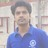#### RajendraSinghRajput2

Aug. 3, 2018
•#### KarunakarKalepelli

Aug. 8, 2020
•#### ssuser901114

Jan. 16, 2021

Total views

2,818

On Slideshare

0

From embeds

0

Number of embeds

4

129

Shares

0